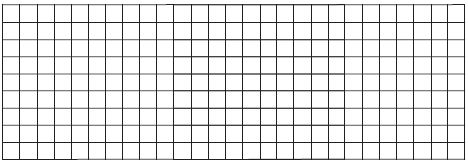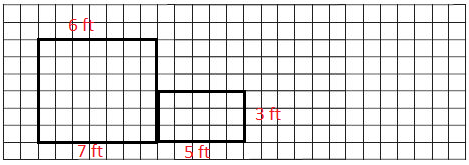# Go Math Grade 3 Answer Key Chapter 11 Perimeter and Area Assessment Test

Go Math Grade 3 Answer Key Chapter 11 contains all the topics of chapter 11 which helps to test the student’s knowledge. Through this assessment test, students can check their knowledge. This assessment test is also helpful for the teachers to know how much a student understood the topics.

This chapter can improve student’s math skills, by referring to the Go Math Grade 3 Answer Key Chapter 11 Perimeter and Area Assessment Test, and with the help of this Go Math Grade 3 Assessment Test Answer Key, students can score good marks in the examination

Chapter 11: Perimeter and Area Assessment Test

### Test – Page 1 – Page No. 111

Question 1.
Find the perimeter of each figure on the grid. Identify the figures that have a perimeter of 16 units. Mark all that apply.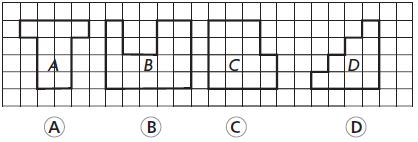Options:
a. A
b. B
c. C
d. D

Answer: a,c,d have a perimeter of 16 units.

Explanation: To find the perimeter we will add the total length of the lines that form a shape.
The perimeter of shape A is 1+4+1+1+1+3+2+3+= 16 units.
The perimeter of shape B is 1+4+5+4+2+2+2+2= 22 units.
The perimeter of shape C is 4+4+2+1+2+3= 16 units.
The perimeter of shape D is 1+1+1+1+1+1+1+4+4+1= 16 units.

Question 2.
Mr. Howard is putting wood trim around his window. How many feet of wood trim does Mr. Howard need for the perimeter of the window?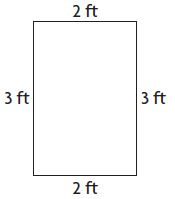_____ feet

Explanation: The perimeter of the window is 3+2+3+2= 10 ft.

Question 3.
Greg drew this figure on dot paper. What is the area of the figure?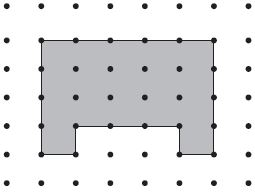_____ square units

Explanation: To find the area we need to count the square units.
So, the area of the figure is 17 square units.

### Test – Page 2 – Page No. 112

Question 4.
Brady is placing square tiles on the floor of the kitchen. Each unit square is 1 square foot.Which equations can Brady use to find the area of the kitchen floor? Mark all that apply.
Options:
a. 4 × 6 = 24
b. 4 + 4 + 4 + 4 + 4 = 20
c. 4 + 6 + 4 + 6 = 20
d. 6 + 6 + 6 + 6 = 24
e. 4 × 5 = 20
f. 6 × 4 = 24

Explanation: The area of the kitchen floor is 6 × 4 = 24  = 20.

Question 5.
Glenda used square tiles to make a rectangle. The rectangle has a perimeter of 8 units and an area of 4 square units. Which could be Glenda’s rectangle?
Options:
a.b.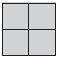c.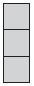d.Explanation: As in the above figures the option d has a perimeter of 8 units and an area of 4 square units.

Question 6.
Jill uses a ruler to draw a square. The perimeter of the square is 20 inches. Select a number to complete the sentence.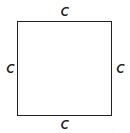The square has a side length of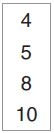inches.
_____

Explanation: As the perimeter of the square is 20 inches. So the side length of the square is
P= 4c
20= 4c
c= 20/4
= 5 inches.

### Test – Page 3 – Page No. 113

Question 7.
Rosa drew two rectangles on grid paper. Circle the words that make the sentence true.Rectangle A has an area that is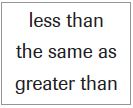area of Rectangle B, and a perimeter that isthe perimeter of Rectangle B.
Area: Rectangle A ________ Rectangle B
Perimeter: Rectangle A __________ Rectangle B

Area: Rectangle A is as same as Rectangle B.
Perimeter: Rectangle A is greater than Rectangle B.

Explanation:
Rectangle A has an area that isarea of Rectangle B and a perimeter that is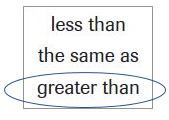the perimeter of Rectangle B.

Question 8.
Ryan drew this figure on grid paper. What is the perimeter of the figure?______ units

Explanation: The perimeter of the figure  is 3+4+1+1+1+1+1+4= 16 units.

Question 9.
What is the area of the figure shown? Each unit square is 1 square foot.______ square feet

Explanation: The area of the figure is 23 square feet.

### Test – Page 4 – Page No. 114

Question 10.
Ella drew a rectangle that was 3 units wide and 4 units long. Draw a different rectangle that has the same perimeter but a different area.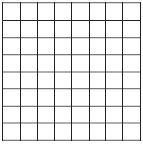Answer: The dimensions are 5 units of length and 2 units of width.

Explanation: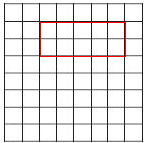First, we will find the perimeter of the given rectangle P=2(L+W)
P= 2(3+4)
= 2(7)
= 14.
Now we want to come up with a different combination of numbers that would give up a perimeter of 14. We know that 14 is divisible by 2 so let’s make out the width 2. If we plug in 2 as the width and 14 as the perimeters, we can solve for length. So
14= 2L+2×2
14= 2L+4
14 – 4 = 2L
10= 2L
L= 5.
So the dimensions are 5 units of length and 2 units of width.

Question 11.
Kendra glued ribbon around the outside border of the shape shown below. She used 30 centimeters of ribbon for the border. What is the unknown side length?b = ______ centimeters

Explanation:
The unknown side length is
6+9+4+4+2+b= 30 cm
25+b= 30
b= 30- 25
b= 5 cm.

Question 12.
A carpenter made two tables. The first table is 4 feet wide and 10 feet long. The second table is half as long as the first table. The area of the second table is one fourth the area of the first table.
For numbers 12a–12d, select True or False.
a. The width of the second table is 2 feet.
i. True
ii. False

Explanation: As the second table is half as long as the first table, so the wide of the second table is 2 feet.

Question 12.
b. The area of the second table is 10 square feet.
i. True
ii. False

Explanation:
The area of the first table is 4×10= 40 square feet.
As the area of the second table is one fourth the area of the first table, so the area of the second table is 1/4×40= 10 square feet.

Question 12.
c. The length of the second table is 8 feet.
i. True
ii. False

Explanation: The length of the second table is 5 feet.

Question 12.
d. The area of the first table is 28 square feet.
i. True
ii. False

Explanation: The area of the first table is 40 square feet.

### Test – Page 5 – Page No. 115

Question 13.
Fiona bought some pictures. Each picture had a perimeter of 24 inches. Which could be one of the pictures Fiona bought? Mark all that apply.
Options:
a.b.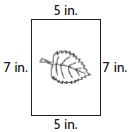c.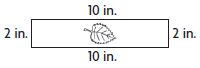d.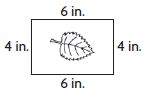Explanation:
The perimeter of figure b is 7+5+7+5= 24 in.
The perimeter of figure c is 2+10+2+10= 24 in.

Question 14.
Tasha wants to fence in two different rectangular pens for her chickens, each with an area of 36 square feet. She will buy fencing to build each pen.
Part A
Each unit square on the grid below is 1 square foot. Draw two possible pens. Label each with a letter.Answer: The two rectangles with 36 square feet each are drawn below.

Explanation:
A                                      B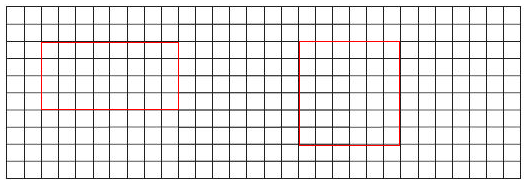Question 14.
Part B
Which of the pens will take more fencing to make?
Explain how you know.

Answer: Pen A will take more time for fencing.

Explanation: Pen A perimeter is 4+9+4+9= 26 feet and pen B perimeter is 6+6+6+6= 24 feet. So the pen A will take more time for fencing.

### Test – Page 6 – Page No. 116

Question 15.
Simon draws a sketch of the floor of his tree house on grid paper. Each unit square is 1 square foot. Write and solve a multiplication equation that can be used to find the area of the floor in square feet.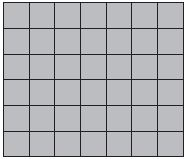_____ square feet

Explanation: The multiplication equation of the area of the floor in square feet is 6×7= 42 square feet.

Question 16.
Rachel uses grid paper to plan a mural to paint at her school. The design will be made of two connected rectangles. The larger rectangle will have an area between 35 square feet and 45 square feet. The smaller rectangle will have an area between 10 square feet and 20 square feet. Draw and label a diagram to show what Rachel could plan. Explain how to find the total area.